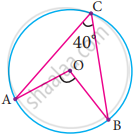Tamil Nadu Board of Secondary EducationSSLC (English Medium) Class 9th

# In the figure, O is the centre of the circle and ∠ACB = 40° then ∠AOB = - Mathematics

MCQ

In the figure, O is the centre of the circle and ∠ACB = 40° then ∠AOB =• 80°

• 85°

• 70°

• 65°

#### Solution

80°

Explanation;

Hint:

∠AOB = 2∠ACB ...(The angle subtended by an arc of the circle at the centre is double the angle subtended at the remaining part of the circle.)

= 2 × 40°

= 80°

Concept: Theorem: The angle subtended by an arc of the circle at the centre is double the angle subtended by it at any point on the remaining part of the circle.
Is there an error in this question or solution?